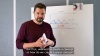# Video 5: Implementing the Formula### 🎥 Video 5Implementing the Formula

Today we show you how to implement the formula.

One of the key components to successfully implementing this formula is using ScoreApp!

Implementing the Formula
Implementing the Formula
5/5

Video 5
Implementing the Formula

📋 Exercise 5

### Implementing the Formula

Exercise 1

Write down, in the last 4 weeks:

How many appointments got set?

How many times did you or

How many sale?

Exercise 2